# Gas/Water Interfacial Tension

Jump to: navigation, search

## Gas/Water Interfacial Tension

Gas/Water Interfacial Tension correlation was published by Baker and Swerdloff in 1956.

## Math and Physics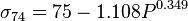$\sigma_{74} =75 - 1.108P^{0.349}$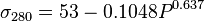$\sigma_{280} =53-0.1048P^{0.637}$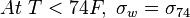$At\ T<74F,\ \sigma_w=\sigma_{74}$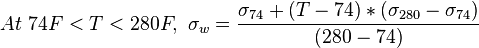$At\ 74F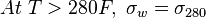$At\ T>280F,\ \sigma_w=\sigma_{280}$

## Nomenclature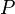$P$ = pressure, psia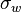$\sigma_w$ = gas/water interfacial tension, dynes/cm$T$ = temperature, °F

## See also

Gas/Oil Interfacial Tension
Gas/Water Interfacial Tension
Water bubble point pressure
Water compressibility
Water density
Water formation volume factor
Water salinity from density equation
Water solids concentration
Water viscosity